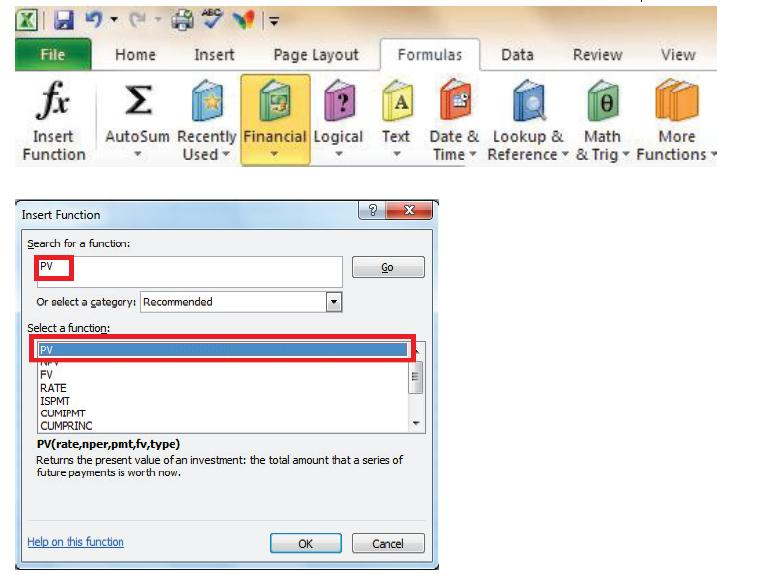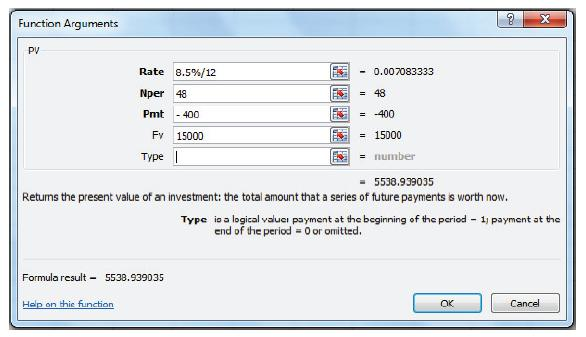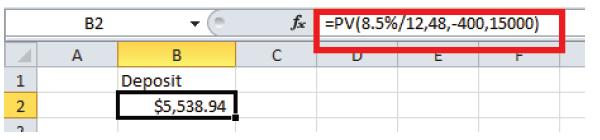# Business Automation and Software Blog

Excel Tips and Tricks / Business Reporting Question: How much deposit do I need to pay in order to have a monthly installment of \$400 over a period of 4 years for a car costing \$15,000? The interest rate is 8.5%.

Answer: The PV function will be used to find a solution. The PV Function returns the present value of an investment. The present value is the total amount that a series of future payments is worth now. For example, when you borrow money, the loan amount is the present value to the lender.

Why: To calculate the amount of deposit to be paid for a car costing \$15,000

Applies To: Excel 2010, 2007, and 2003

1. Select Formulas- Insert function > Financial and select as below:2. Select Ok and enter as below:3. Select OK.

The amount of deposit to be paid for a car costing \$15 ,000 at a monthly installment of \$400 over 4 years and interest rate of 8.5% is \$ 5 538.94 . You can also type the formula in excel as; =PV(8.5%/12,48,- 400,15000).PV(rate, nper, pmt, [fv], [type])

The PV function syntax has the following arguments (argument: A value that provides information to an action, an event, a method, a property, a function, or a procedure):

• Rate Required. The interest rate per period. For example, if you obtain an automobile loan at a 10 percent annual interest rate and make monthly payments, your interest rate per month is 10%/12, or 0.83%. You would enter 10%/12, or 0.83%, or 0.0083, into the formula as the rate.
• Nper Required. The total number of payment periods in an annuity. For example, if you get a four-year car loan and make monthly payments, your loan has 4*12 (or 48) periods. You would enter 48 into the formula for nper.
• Pmt Required. The payment made each period and cannot change over the life of the annuity. Typically, pmt includes principal and interest but no other fees or taxes. For example, the monthly payments on a \$10,000, four-year car loan at 12 percent are \$263.33. You would enter -263.33 into the formula as the pmt. If pmt is omitted, you must include the fv argument.
• Fv Optional. The future value or a cash balance you want to attain after the last payment is made. If fv is omitted, it is assumed to be 0 (the future value of a loan, for example, is 0). For example, if you want to save \$50,000 to pay for a special project in 18 years, then \$50,000 is the future value. You could then make a conservative guess at an interest rate and determine how much you must save each month. If fv is omitted, you must include the pmt argument.
• Type Optional. The number 0 or 1 and indicates when payments are due.